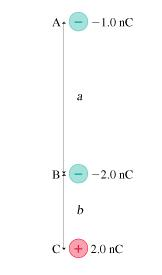Home / Answered Questions / Other / 1-what-is-the-magnitude-of-the-net-electric-force-on-charge-b-in-the-figure-figure-1-assume-aaa-2-0--aw623

# (Solved): 1. What Is The Magnitude Of The Net Electric Force On Charge B In The Figure?(Figure 1) Assume Aaa =...1.

What is the magnitude of the net electric force on charge B in the figure?(Figure 1) Assume aaa = 2.0 cmcm and bbb = 0.70 cmcm .

Express your answer to two significant figures and include the appropriate units.

2.

What is the direction of the net electric force on charge B in the figure?

 The force is directed up. The force is directed down.
A- -1.0 nC B: -2.0 nC c + 2,0 nC

We have an Answer from Expert